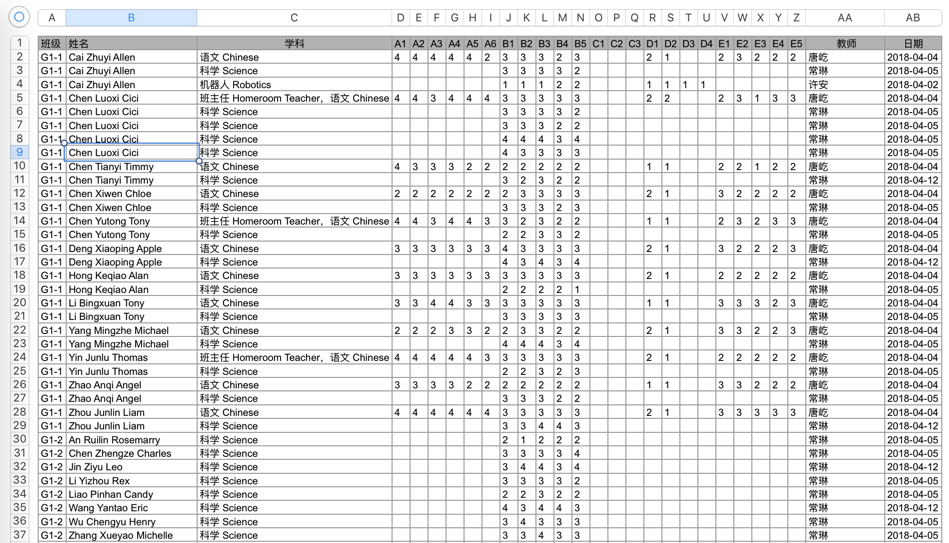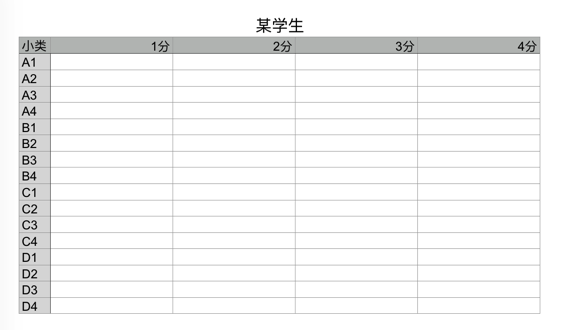【SQL】求统计查询的SQL语句 80C

===== 4 月 9 日下午 4 点补充说明 =====15个回答

......

SELECT * FROM (SELECT ID,A1,A2,A3,A4 FROM 评分表 GROUP BY ID,A1,A2,A3,A4 ) A UNION
SELECT * FROM (SELECT ID,B1,B2,B3,B4 FROM 评分表 GROUP BY ID,B1,B2,B3,B4 ) B UNION
SELECT * FROM (SELECT ID,C1,C2,C3,C4 FROM 评分表 GROUP BY ID,C1,C2,C3,C4 ) C UNION
SELECT * FROM (SELECT ID,D1,D2,D3,D4 FROM 评分表 GROUP BY ID,D1,D2,D3,D4 ) D

A1~A4每个都要评分，还是说A1就是1分，A2就是2分；A,B,C,D代表4个科目？每个科目教师为一个元组？一个学生需要4个元组。

SQL如下：

create table testxzq (bnid varchar2(50),
classnum int,studentid varchar2(50),sourceid varchar2(50),teacherid varchar2(50),
A1 number,A2 number ,B1 number,B2 number,c1 number ,c2 number
);

select t.studentid,t.sourceid,
sum(nvl(t.a1,0)+nvl(t.a2,0)) A,sum(nvl(t.b1,0) + nvl(t.b2,0)) B,sum(nvl(t.c1,0)+nvl(t.c2,0)) C
from testxzq t
group by t.studentid,t.sourceid;topshow_2010 这个是取分数的第一步，然后根据分数分段取各个分数的人数

select sid,(count(A1) + count(A2) + count(A3) + count(A4)) as a , (count(B1) + count(B2) + count(B3) + count(B4)) as b,(count(C1) +count(C2) + count(C3) + count(C4)) as c , (count(D1) + count(D2) + count(D3) + count(D4)) as d from table

select d.sourceid,
sum(case when d.a = 1 then 1 else 0 end ) A1,
sum(case d.a when 2 then 1 else 0 end ) A2,
sum(case d.a when 3 then 1 else 0 end ) A3,
sum(case d.a when 4 then 1 else 0 end ) A4,
sum(case d.b when 1 then 1 else 0 end ) B1,
sum(case d.b when 2 then 1 else 0 end ) B2,
sum(case d.b when 3 then 1 else 0 end ) B3,
sum(case d.b when 4 then 1 else 0 end ) B4,
sum(case d.c when 1 then 1 else 0 end ) C1,
sum(case d.c when 2 then 1 else 0 end ) C2,
sum(case d.c when 3 then 1 else 0 end ) C3,
sum(case d.c when 4 then 1 else 0 end ) C4
from (
select t.studentid,t.sourceid,
sum(nvl(t.a1,0)+nvl(t.a2,0)) a,sum(nvl(t.b1,0) + nvl(t.b2,0)) b,sum(nvl(t.c1,0)+nvl(t.c2,0)) c
from testxzq t
group by t.studentid,t.sourceid
) d group by d.sourceid
;

if (object_id('tgr_update', 'TR') is not null)

drop trigger tgr_update
go
create trigger tgr_clasupdate' B

for update
as

declare @A1 int, @newB1 int;
--更新前的数据

select @oldnewB1=b1,@A1=a1 From inserted

if (@olnewB1==1)

begin
--更新后的数据

update A set A2= 1 where A1 = @A1

end

go

--表名： b_test,假设A1-D4字段类型是字符如果是数字则先转字符,并且不会为空如果为空则去空为0
--SQL思路就是先将每条评分记录连成字符串，再从中取各评分字符的出现次数，最后将不同教师不同评分个数合计

select 班级,学生,SUM(LENGTHB(TRANSLATE(合计,'1'||合计,'1'))) 1分个数,
SUM(LENGTHB(TRANSLATE(合计,'2'||合计,'2'))) 2分个数,
SUM(LENGTHB(TRANSLATE(合计,'3'||合计,'3'))) 3分个数,
SUM(LENGTHB(TRANSLATE(合计,'4'||合计,'4'))) 4分个数
from (select 班级,学生,学科,教师,A1||A2||A3||A4||B1||B2||B3||B4||C1||C2||C3||C4||D1||D2||D3||D4 合计 from b_test )
group by 班级,学生NET_NDSC_WZP 这个是ORACLE 语法的写法,如果是其他数据库可以按上面的思路修改SQLaccess sql语句 数据查询

MySql执行分页时查询统计数据量的Sql语句差别及性能

sql语句判断查询

sql语句查询，最大最小的问题

sql语句 需要统计个数

select t.DICT_NAME from T_HZDRP_SYS_DICT t WHERE t.PARENT_ID='HZ2883575c8b3d04015c8b53483c0003' 查询所有的职称 SELECT h.expert_title from h_bas_expert h 查询员工职称 需要查询出来 所有的职称对应的个数 需要考虑为0的 不是SELECT COUNT (a.id) AS COUNT,a.expert_title FROM h_bas_expert a GROUP BY a.expert_title只统计员工职称个数

sql查询语句sql联合查询

SQL 语句怎么查询某个时间段内的数据

begintime是开始时间，endtime是结束时间。**这两个是条件**。<br> 想要实现，这两个条件有值，或者其中一个条件有值就按条件查询，<br> 没有条件就查询全部。<br> 求sql语句怎么写，用的sqlserver

sqlserver查询数据库权限的sql语句

sql语句查询总数，每天对应一个数字

web后台的sql查询语句问题

C#使用sql查询语句求解

sing System; using System.Data; using System.Data.SqlClient; using System.Collections.Generic; using System.Linq; using System.Windows.Forms; namespace library { class linksql { public void con(string shuming) { string connString; connString = "Data Source=DESKTOP-MPLGIDU\\SQLEXPRESS;uid=sa;pwd=admin;database=book;"; SqlConnection sConn = new SqlConnection(connString); try { sConn.Open(); } catch (Exception ex) { Console.WriteLine("连接错误:" + ex.Message); } string sql; sql = "declare @cry varchar(20) set @cry=shuming select * from book1 where 书名=@cry"; SqlCommand sCmd = new SqlCommand(sql, sConn); SqlDataReader sdr = null; sdr = sCmd.ExecuteReader(); while (sdr.Read()) { Console.WriteLine(sdr + " " + sdr["书名"] + sdr["作者"] + " " + sdr["索书号"]); } sdr.Close(); sConn.Close(); } } }

sql查询语句怎么写????

oracle sql语句统计数据写法

PLsql 执行sql语句查询超时

sql查询语句中字段有几十个，并且有大量的聚合函数，求最大最小值，求和之类的 ，表中数据量非常多，也建了索引，在PLsql 中执行sql语句，一直在执行，强行停止停止不下来， 怎么处理，sql语句怎么优化，数据库是不是有点问题啊

SQL统计查询在C# winfor前台显示

SQL 统计查询 下面的SQL语句，只有单价，需要统计费用次数和费用总价 ``` select a.CType as '类别',a.ChargeDetailsName as '费用名称',a.CDPayServiceName as '收费项目', a.ChargeDetailsPrice as '费用单价', a.CDExecutiveSectionName as '执行科室', a.CDOpenBillName as '开单科室',a.CDTime as '记账时间',a.CDHopitalName as '医院名称', b.StaffName as '开单医生',c.CFRemarks as '费用类别' from HJY_ChargeDetailsInfo as a,HJY_StaffInfo as b,HJY_ChargeClassificationInfo as c where a.SID = b.SID and a.CDPayServiceName = c.ChargeClassificationName ```

loonggg读完需要3分钟速读仅需 1 分钟大家好，我是你们的校长。我之前讲过，这年头，只要肯动脑，肯行动，程序员凭借自己的技术，赚钱的方式还是有很多种的。仅仅靠在公司出卖自己的劳动时...

MySQL数据库面试题（2020最新版）

!大部分程序员只会写3年代码

HTTP与HTTPS的区别